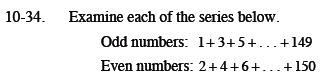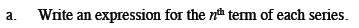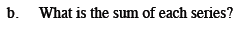Home > CCA2 > Chapter 10 > Lesson 10.1.2 > Problem10-34

10-34.
1. Examine each of the series below. Homework Help ✎
Odd numbers: 1 + 3 + 5 + … + 149
Even numbers: 2 + 4 + 6 + … + 150

1. Write an expression for the nth term of each series.

2. What is the sum of each series?t(n) = 1 + 2(n − 1)
t(n) = 2 + 2(n − 1)Use the rectangle method or Antonio's method.
Both series have 75 terms.